NOTE: This section is Under-Edit if necessary: Construction began on August 13, 2013 and finished on September 17, 2013.

Soft-Decision Outputs Channel Decoding (SOVA & 'Symbol-by-Symbol' MAP Algorithms) of Convolutional Coded Signaling over a Coherent Memoryless Channel

The AdvDCSMT1DCSS (T1) Professional (T1 Version 2) system tool provides the capability to model and simulate Single Iteration Soft-Decision Output (SO) Convolutional Code (CC) Channel Decoding using a SO Viterbi Algorithm (SOVA) or a 'symbol-by-symbol' Maximum a Posteriori Probability (MAP) Algorithm. These decoding algorithms can be used in the cases for Convolutional Coded Signaling over Memoryless Channels (MLC) with Additive White Gaussian Noise (AWGN). Three MAP Decoding Algorithms (Max-Log-MAP, Log-MAP, and MAP) are supported by T1 V2. Note that an Information Bit Interleaver and Soft-Output DeInterleaver are used in this new feature. T1 V2 provides the User the ability to select a Block, Pseudo-Random, Quadratic Permutation Polynomial, or Identity Interleaver-DeInterleaver type. Also, this capability of T1 Professional supports decoding of CC Signaling over a Noiseless or BSC MLC, too.

The SOVA and MAP algorithms development in T1 V2 was aided by the study of their descriptions in a number of important works. The following key works: six journal and conference papers and one book will provide an important background information about Soft-Decision Outputs Decoding:

1) Joachim Hagenauer and Peter Hoeher, "A Viterbi Algorithm with Soft-Decision Outputs and its Applications," in Proc. of IEEE GLOBECOM, Dallas, TX, pp. 1680-1686, November 1989.

2) Joachim Hagenauer, Elke Offer, and Lutz Papke, "Iterative Decoding of Binary Block and Convolutional Codes," IEEE Transactions on Information Theory, Vol. 42, No. 2, pp. 429-445, March 1996.

3) Shu Lin and Daniel J. Costello, Jr., Error Control Coding: fundamentals and applications, Second Edition, Pearson Prentice Hall, Upper Saddle River, New Jersey, 2004.

4) Andrew J. Viterbi, "An Intuitive Justification and a Simplified Implementation of the MAP Decoder for Convolutional Codes," IEEE Journal on Selected Areas in Communications, Vol. 16, No. 2, pp. 260-264, February 1998.

5) L.R. Bahl, J. Cocke, F. Jelinek, and J. Raviv, "Optimal Decoding of Linear Codes for Minimizing Symbol Error Rate," IEEE Transactions on Information Theory, Vol. IT-20, No. 2, pp. 284-287, March 1974.

6) Patrick Robertson, Emmanuelle Villebrun, and Peter Hoeher, "A Comparison of Optimal and Sub-Optimal MAP Decoding Algorithms Operating in the Log Domain," in Proc. IEEE ICC'95, Seattle, WA, pp. 1009-1013, 1995.

7) G. David Forney, Jr., "The Viterbi Algorithm," Proceedings of the IEEE, Vol. 61, No. 3, pp. 268-278, March 1973.

Consider the SOVA and MAP Algorithm Bit Error Rate (BER) or Bit Error Probability Pb performance simulation results that were produced by T1 V2 that are displayed below in Figure 1, 3, and 4 plots. Also, a Peak Channel Symbol Signal-to-Noise Ratio (SNR) Es/N0 plot corresponding to these results are displayed below in Figure 2. In addition, BER and Peak Channel Symbol Signal-to-Noise Ratio (SNR) results are presented for UnCoded Signaling over a Coherent MLC with AWGN. Note that CC BER results are based on the simulated transmission of 10,000,384 Information Bits. For the UnCoded cases, BER results are based on the simulated transmission of 10,000,002 Information Bits.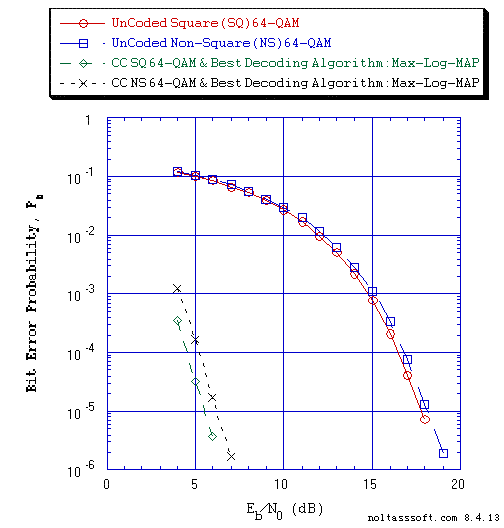```Figure 1. Bit Error Probability for UnCoded and Rate = 1/6 Convolutional Coded (CC)
Square and Non-Square 64-QAM Signaling over a Coherent Memoryless Channel
with Additive White Gaussian Noise (AWGN):
```
```          Equal probable IID Source for 10,000,002 Information Bits for UnCoded
64-QAM Signaling over a Vector Channel;```
```          Equal probable IID Source for 10,000,384 Information Bits for Convolutional
Coded 64-QAM Signaling over a Vector Channel;
```
```          Rate = 1/6, K = 4, (63, 60, 51, 63) a Best (Optimal) Non-Recursive
Convolutional Code;```
`          Square 64-QAM signal constellation with Gray encoding;`
`          Non-Square 64-QAM signal constellation with 'Impure' Gray encoding;`
```          1024-Information Bit Identity Interleaver and 1024-Soft-Decision Value
Identity DeInterleaver; &```
```          Max-Log-MAP (Maximum a Posteriori Probability) Algorithm
('Symbol-by-Symbol') Channel Decoder using an Unquantized Branch Metric.```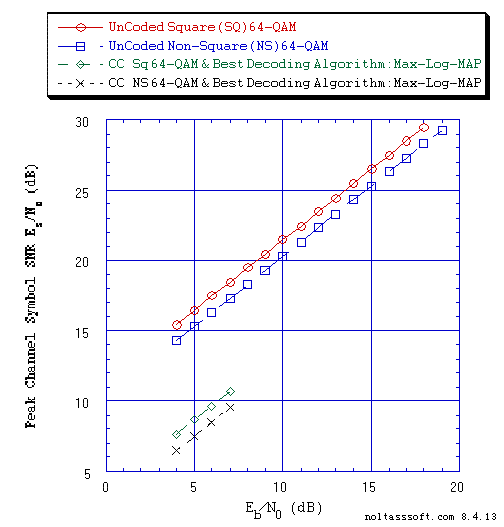```Figure 2. Peak Channel Symbol Signal-to-Noise Ratio (SNR), Es/N0 for UnCoded and
Rate = 1/6 Convolutional Coded(CC) Square and Non-Square 64-QAM Signaling
over a Coherent Memoryless Channel with Additive White Gaussian Noise
(AWGN):
```
```          Equal probable IID Source for 10,000,002 Information Bits for UnCoded
64-QAM Signaling over a Vector Channel;```
```          Equal probable IID Source for 10,000,384 Information Bits for
Convolutional Coded 64-QAM Signaling over a Vector Channel;```
```          Rate = 1/6, K = 4, (63, 60, 51, 63) a Best (Optimal) Non-Recursive
Convolutional Code;```
`          Square 64-QAM signal constellation with Gray encoding;`
`          Non-Square 64-QAM signal constellation with 'Impure' Gray encoding;`
```          1024-Information Bit Identity Interleaver and 1024-Soft-Decision Value
Identity DeInterleaver; &```
```          Max-Log-MAP (Maximum a Posteriori Probability) Algorithm
('Symbol-by-Symbol') Channel Decoder using an Unquantized Branch Metric.```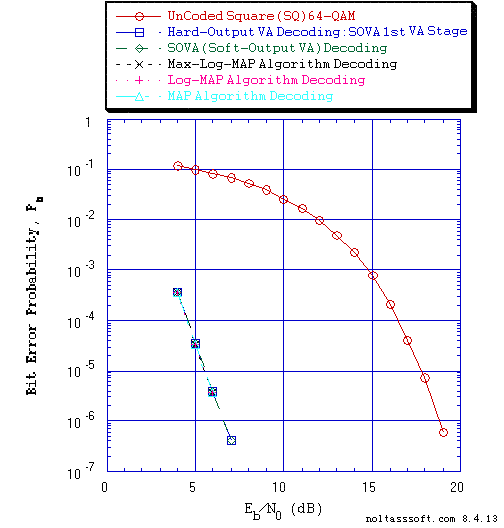```Figure 3. Bit Error Probability for UnCoded and Rate = 1/6 Convolutional Coded
Square 64-QAM Signaling over a Coherent Memoryless Channel with
```
```          Equal probable IID Source for 10,000,002 Information Bits for UnCoded
64-QAM Signaling over a Vector Channel; &
```
```          Equal probable IID Source for 10,000,384 Information Bits for
Convolutional Coded 64-QAM Signaling over a Vector Channel.```
```          Rate = 1/6, K = 4, (63, 60, 51, 63) a Best (Optimal) Non-Recursive
Convolutional Code;```
`          Square 64-QAM signal constellation with Gray encoding;`
```          1024-Information Bit Identity Interleaver and 1024-Soft-Decision Value
Identity DeInterleaver;```
```          Soft-Output Viterbi Algorithm (SOVA) Decoder (Two Stage) using a Path
Memory Length of 20 bits and an Unquantized Branch Metric;```
`          SOVA 1st Stage VA Decoder: Hard-Decision Output VA; &`
```          Max-Log-MAP, Log-MAP, and MAP (Maximum a Posteriori Probability) Algorithm
('Symbol-by-Symbol') Channel Decoder using an Unquantized Branch Metric.```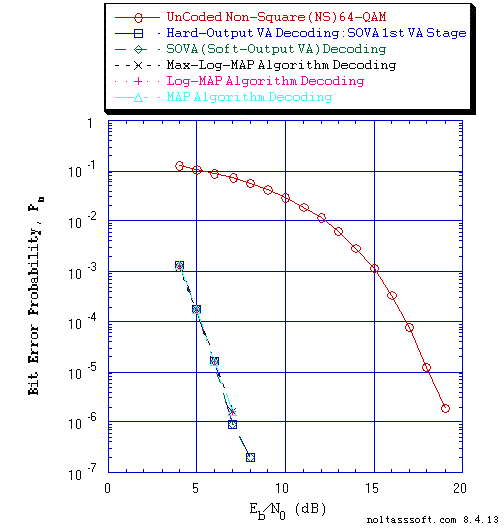```Figure 4. Bit Error Probability for UnCoded and Rate = 1/6 Convolutional Coded
Non-Square 64-QAM Signaling over a Coherent Memoryless Channel with
```
```          Equal probable IID Source for 10,000,002 Information Bits for
UnCoded 64-QAM Signaling over a Vector Channel;
```
```          Equal probable IID Source for 10,000,384 Information Bits for
Convolutional Coded 64-QAM Signaling over a Vector Channel;```
```          Rate = 1/6, K = 4, (63, 60, 51, 63) a Best (Optimal) Non-Recursive
Convolutional Code;```
`          Non-Square 64-QAM signal constellation with 'impure' Gray encoding;`
```          1024-Information Bit Identity Interleaver and 1024-Soft-Output Value
Identity DeInterleaver;```
```          Soft-Decision Output Viterbi Algorithm (SOVA) Decoder (Two Stage)
using a Path Memory Length of 20 bits and an Unquantized Branch Metric;```
`          SOVA 1st Stage VA Decoder: Hard-Decision Output VA; &`
```          Max-Log-MAP, Log-MAP, and MAP (Maximum a Posteriori Probability) Algorithm
('Symbol-by-Symbol') Channel Decoder using an Unquantized Branch Metric.```
To evaluate this new T1 Professional feature of SO Channel Decoding, the modulation type M-QAM (M-ary Quadrature Amplitude Modulation) was chosen because it allows for the use of interesting signal vector spaces (constellations), i.e., Square versus Non-Square distribution of signal vectors in M-QAM 2-D signal space. Thus, 'impure' Gray coded Non-Square (NS) 64-QAM and the Gray coded Square (SQ) 64-QAM modulation were chosen for testing this new T1 feature. The Non-Square constellation was constructed by modifying the Square constellation by relocating each quadrant's two signal vectors (innermost and corner) to specific available locations (signal vector position near the I axis & signal vector position next to the Q axis, respectively) that would result in a reduced Peak Channel Symbol Signal-to-Noise Ratio (SNR) during signaling. Consult Figure 5 for a depiction of this NS 64-QAM constellation.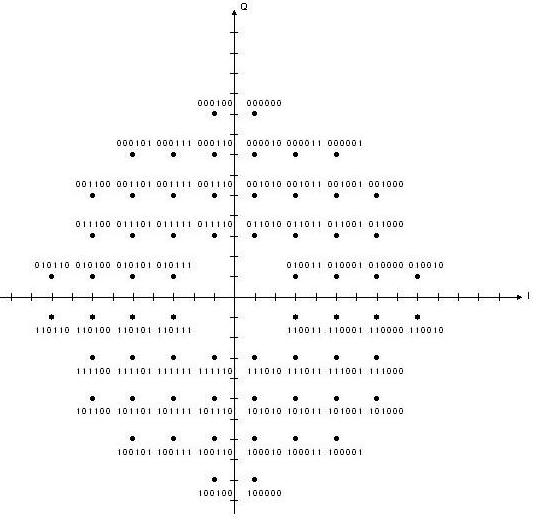```Figure 5. 'Impure' Gray Coded Non-Square 64-QAM Signal Vector Space (constellation).
```
For Convolutional Coded signaling with the 64-QAM modulation scheme, the Rate R = 1/6, Constraint Length K = 4 code [Best (Optimal) Non-Recursive] was chosen.

Now, it is important to note that an Information Bit Identity Interleaver of length 1024 bits and Soft-Input SO Identity DeInterleaver of 1024 values were used for each SOVA, Max-Log-MAP, Log-MAP, and MAP Decoding simulation case. The Identity Interleaver-DeInterleaver performs no permutation of the inputted values. This Identity Interleaver-DeInterleaver allows for the sampling of the Hard-Decision Output (HO) taken from the first Viterbi Algorithm (VA) decoder in the two-stage SOVA decoding structure. This HO (Estimated Transmitted Bit) is used to calculate a reference BER. It is important to note that the use of an Interleaver-DeInterleaver in the Simulated System (SS) changes the Random Processes realization as seen by the SS as compared to the Simulated System without an Interleaver-DeInterleaver.

There are many important conclusions that can be drawn from the above displayed SOVA and MAP Decoding simulated results. It appears that T1 V2 is correctly modeling and simulating the SO (SOVA and MAP Algorithm) Channel Decoders. Also, it appears that HOVA, SOVA, and MAP Algorithms (Max-Log-MAP, Log-MAP, MAP) decoding provide essentially the same decoding performance at relatively low Eb/N0. But Max-Log-MAP decoding provides the best performance for both SQ and NS 64-QAM signaling.

And the NS 64-QAM versus SQ 64-QAM does provide an interesting advantage, i.e., the reduction in Peak Channel Symbol SNR dB is greater than the increase in Eb/N0 dB (at BER = 1.0e-5) for NS 64-QAM as compared to SQ 64-QAM:
```1) 0.77 dB advantage for UnCoded 64-QAM signaling; &
2) 0.46 dB advantage for Convolutional Coded 64-QAM.
```
T1 Professional (T1 Version 2) now offers two fundamental different types of CC Channel Decoding algorithms to the User: the Viterbi Algorithm and the 'symbol-by-symbol' MAP algorithm. These decoding algorithms differ in complexity and type of output (Hard-Decision and Soft-Decision). The User via T1 V2 can get experience with these SO algorithms as applied to Non-Iterative Decoding in simulated digital communication systems prior to their use in Iterative Decoding of Turbo Coded Signals applications.

```BUY T1 Version 2 (ADVDCSMT1DCSS Professional software system tool)NOW.
```